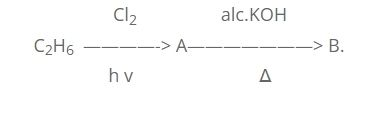# NEB Model Question for Chemistry – Class 11

NEB QUESTION MODEL OF CHEMISTRY
FOR : 2070

Exam Oriented Model Question
Time : 3 hrs

Full Marks : 75

Pass Marks : 27
Group ‘A’

Attempt any Fifteen question : 15 × 2 = 30

Q.1. State Law of reciprocal proportion.

Q.2. The oxide of an element contains 67.67 % of oxygen and V.D. of its volatile chloride is 79

Q.3. Define surface tension of Liquid.

Q.4. Write down important differences between crystalline and amorphous solid.

Q.5. What is Pauli’s exclusion principle ?

Q.6. Give the values of all four quantum numbers of 11th electron of magnesium.(At. No. 12)

Q.8. Write the Lewis structure of (a) H2O2 and (b) HNO3.

Q.9. Explain why HCL has polar character though it has covalent bond.

Q.10. State Modern Periodic law.

Q.11. How would you show the following reaction is a redox reaction?
Mg + Cl2 —————> MgCl2

Q.12. State Le-Chateliers principles.

Q.13. What are the difference between nascent and molecular hydrogen ?

Q.14. name any two oxides of each of the following :
(i) amphoteric
(ii) neutral.

Q.15 What is allotropy ? Name the latest discover allotropic form of carbon.

Q.16. What is meant by acid rain ? Give one major effect of acid rain.

Q.17. Distinguish between flux and slag with one example of each.

Q.18. Can sodium be extracted by the electrolysis of aqueous solutions of sodium chloride ? If not why ?

Q.19. Define electrophile and nucleophile with an example of each.

Q.20. Give IUPAC name of the following compound.
a) CH3-CH(Br)-CH(NH2)-COOH
b) CH3-C(CH3)2-C9OH)H-CH3

Q.21. What is meant by thermal cracking and catalytic cracking ?

Q.22. Identify A and B in the following reaction and give their IUPAC name.Group ‘B’

Attempt any five Question. 5 * 5 = 25

Q.23. Urea [(NH2)2CO] is prepared by reacting ammonia with carbon dioxide :
2NH3(g) + CO2(g) —–> (NH2)2CO(aq.) + H2O(l)
In one process, 637.2 g NH3 is treated with 1142g of CO2.
(a) Which of the following reactant is the limiting reactant ?
(b) Calculate the mass of urea formed
(c) How much excess reagent (in gram) is left at the end of the reaction ?
Q.24 State Avogadro’s law. Using the law to deduce relationship between Molecular mass and vapour density.
Q.25. State and explain Hund’s rule of maximum multiplicity.
Q.26. Specify Oxidation half, reduction half, oxidizing agent and reducing agent. Balance the following equation by ion-electron or oxidation number method :
Fe2+ + H+ + NO3– ——–> Fe+3 + NO + H2O
Q.27. Describe the manufacture of nitric acid by Ostwald’s process.
Q.28. Explain the laboratory preparation of carbon monoxide in laboratory What happens when CO is passed through finely divided nickel ?
Q.29. Describe the detection of foreign element (N,S,X) in organic compounds.

Group ‘C’

Attempt any two questions. : 2 × 10 = 20

Q.30. State Boyle’s law and Charles law. Derive PV=nRT. What is the density (in gram per liter) of ammonia at STP if the gas in a 1.0L bulb weights 0.672g at 25oC and 733.4 mm Hg pressure.

Q.31 Describe the manufacture of sodium carbonate by ammonia soda process. Also, mention the function of limestone in the manufacture process.

Q.32. Write down the process involved in the manufacture of caustic soda by Solvay-Kellner’s process.